Examples of a parameterized surface with embedded curves

Create an example function that returns a position {x, y, z} as a function of two parameters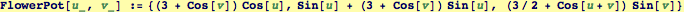Visualize it.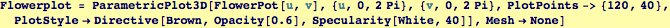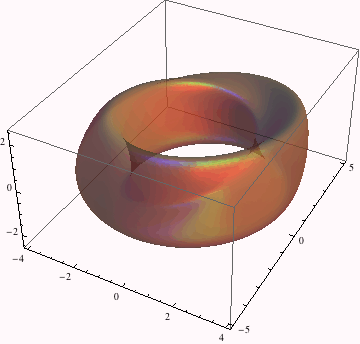A Curve on a parameritized surface

Now, we call the function again, but make the two parameters {u,v}, depend on a single parameter t (*note when visualizing this curve, it has been scaled in and out a little so it will be visible in subsequent visualizations*)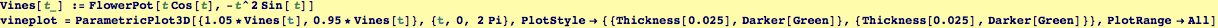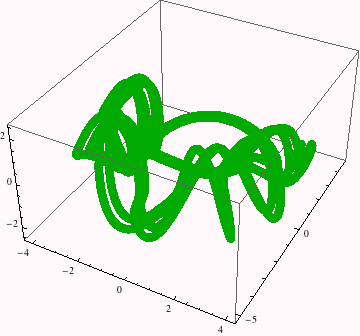This is the paramertized surface with a curve embedded in the surface.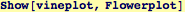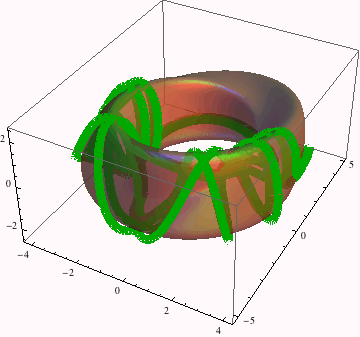Created by Wolfram Mathematica 6.0  (20 September 2007)